Courses

NCERT Exemplar Solutions: Practical Geometry Symmetry & Visualising Solid Shapes Notes | Study Mathematics (Maths) Class 7 - Class 7

Class 7: NCERT Exemplar Solutions: Practical Geometry Symmetry & Visualising Solid Shapes Notes | Study Mathematics (Maths) Class 7 - Class 7

The document NCERT Exemplar Solutions: Practical Geometry Symmetry & Visualising Solid Shapes Notes | Study Mathematics (Maths) Class 7 - Class 7 is a part of the Class 7 Course Mathematics (Maths) Class 7.
All you need of Class 7 at this link: Class 7

Exercise Page: 364

In each of the Questions 1 to 26, there are four options, out of which one is correct. Choose the correct one.
Q.1. A triangle can be constructed by taking its sides as:
(a) 1.8 cm, 2.6 cm, 4.4 cm
(b) 2 cm, 3 cm, 4 cm
(c) 2.4 cm, 2.4 cm, 6.4 cm
(d) 3.2 cm, 2.3 cm, 5.5 cm
Ans: (b)
Explanation: From the rule, a triangle can be constructed when the sum of two sides is greater than the third side.
Consider the length of side 2 cm, 3 cm and 4 cm
2 + 3 = 5, 5 > 4
2 + 4 = 6, 6 > 3
3 + 4 = 7, 7 > 2

Q.2. A triangle can be constructed by taking two of its angles as:
(a) 110o, 40o
(b) 70o, 115o
(c) 135o, 45o
(d) 90o, 90o
Ans: (a)
Explanation: From the rule, sum of all angles of triangle is equal to 180o.
so, 110o + 40o = 150o
and the third angle = 30o

Q.3. The number of lines of symmetry in the figure given below is: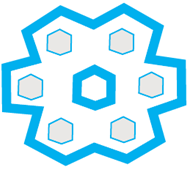(a) 4
(b) 8
(c) 6
(d) Infinitely many
Ans: (c)
Explanation: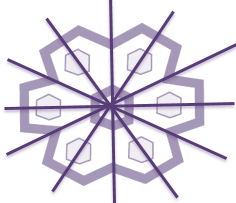A figure has line symmetry, if there is a line about which the figure may be folded so that the two parts of the figure will coincide with each other.

Q.4. The number of lines of symmetry in Fig. 12.14 is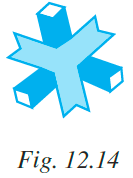(a) 1
(b)
3
(c)
6
(d)
Infinitely many
Ans:
(b)

Q.5. The order of rotational symmetry in the Fig. 12.15 given below is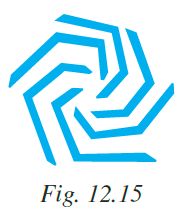(a) 4
(b) 8
(c) 6
(d) Infinitely many
Ans:
(c)
Explanation: In a complete turn (of 360°), the number of times the figure coincides with its original position is called its order of rotational symmetry.

Q.6. The order of rotational symmetry in the figure 12.16 given below is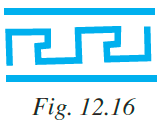(a) 4
(b) 2
(c) 1
(d) Infinitely many
Ans: (b)
Explanation: In a complete turn (of 360°), the number of times the figure coincides with its original position is called its order of rotational symmetry.

Q.7. The name of the given solid in Fig 12.17 is: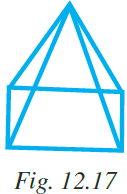(a) triangular pyramid
(b) rectangular pyramid
(c) rectangular prism
(d) triangular prism
Ans:
(b)
Explanation: The given figure contains rectangle base and triangle sides. Therefore, the given figure is the combination of rectangle and triangle called rectangular pyramid.

Q.8. The name of the solid in Fig. 12.18 is: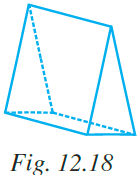(a) triangular pyramid
(b) rectangular prism
(c) triangular prism
(d) rectangular pyramid
Ans: (c)
Explanation: The given figure contains parallelogram faces and triangle sides. Therefore, the given figure is the combination of parallelogram and triangle called triangular prism.

Q.9. All faces of a pyramid are always:
(a) Triangular
(b) Rectangular
(c) Congruent
(d) None of these

Ans: (d)
Explanation: The faces of pyramid can be rectangular and triangular.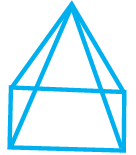Q.10. A solid that has only one vertex is
(a) Pyramid
(b) Cube
(c) Cone
(d) Cylinder

Ans: (c)
Explanation: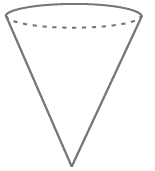Vertex is defined as the common endpoint of two or more rays or line segments. Vertex typically means a corner or a point where lines meet.

Q.11. Out of the following which is a 3-D figure?
(a) Square
(b) Sphere
(c) Triangle
(d) Circle
Ans:
(b) Sphere
Explanation: 3 – Dimensional shape can be defined as solid figure that has three dimensions i.e. length, width and height.

Q.12. Total number of edges a cylinder has
(a) 0
(b) 1
(c) 2
(d) 3

Ans: (c)
Explanation: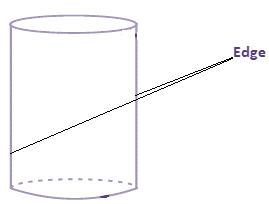Q.13. A solid that has two opposite identical faces and other faces as parallelograms is a
(a) prism
(b) pyramid
(c) cone
(d) sphere

Ans: (a)
Explanation: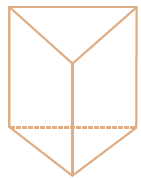Q.14. The solid with one circular face, one curved surface and one vertex is known as:
(a) cone
(b) sphere
(c) cylinder
(d) prism

Ans: (a)
Explanation:Q.15. If three cubes each of edge 4 cm are placed end to end, then the dimensions of resulting solid are:
(a) 12 cm × 4 cm × 4 cm
(b) 4 cm × 8 cm × 4 cm
(c) 4 cm × 8 cm × 12 cm
(d) 4 cm × 6 cm × 8 cm
Ans:
(a)

Q.16. When we cut a corner of a cube as shown in the figure 12.19, we get the cutout piece as: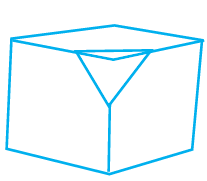(a) square pyramid
(b) trapezium prism
(c) triangular pyramid
(d) a triangle

Ans: (c)

Q.17. If we rotate a right-angled triangle of height 5 cm and base 3 cm about its height a full turn, we get
(a) cone of height 5 cm, base 3 cm
(b) triangle of height 5 cm, base 3 cm
(c) cone of height 5 cm, base 6 cm
(d) triangle of height 5 cm, base 6 cm
Ans: (a)
Explanation: (a) cone of height 5 cm, base 3 cm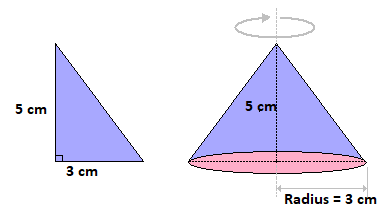Q.18. If we rotate a right-angled triangle of height 5 cm and base 3 cm about its base, we get:
(a) cone of height 3 cm and base 3 cm
(b) cone of height 5 cm and base 5 cm
(c) cone of height 5 cm and base 3 cm
(d) cone of height 3 cm and base 5 cm
Ans:
(d)
Explanation: (d) cone of height 3 cm and base 5 cm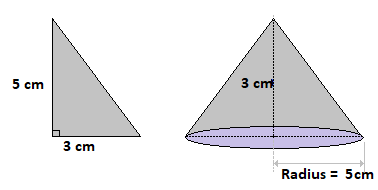Q.19. When a torch is pointed towards one of the vertical edges of a cube, you get a shadow of cube in the shape of
(a) square
(b) rectangle but not a square
(c) circle
(d) triangle
Ans: (b)

Q.20. Which of the following sets of triangles could be the lengths of the sides of a right-angled triangle:
(a) 3 cm, 4 cm, 6 cm
(b) 9 cm, 16 cm, 26 cm
(c) 1.5 cm, 3.6 cm, 3.9 cm
(d) 7 cm, 24 cm, 26 cm
Ans: (c)
Explanation: From the Pythagoras theorem,
Hypotenuse2 = base2 + heihgt2
Consider 3.9cm is the length of hypotenuse.
(3.9)2 = (1.5)2 + (3.6)2
15.21 = 2.25 + 12.96
15.21 = 15.21

Q.21. In which of the following cases, a unique triangle can be drawn
(a) AB = 4 cm, BC = 8 cm and CA = 2 cm
(b) BC = 5.2 cm, ∠B = 90° and ∠C = 110°
(c) XY = 5 cm, ∠X = 45° and ∠Y = 60°
(d) An isosceles triangle with the length of each equal side 6.2 cm.
An: (c)
Explanation: A triangle can be drawn if two angles and a side measurement are given.

Q.22. Which of the following has a line of symmetry?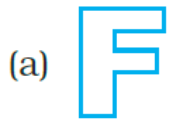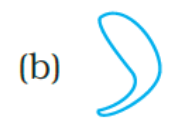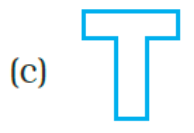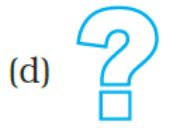Ans: (c)
Explanation: Figure (c) has a line of symmetry.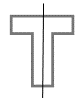A figure has line symmetry, if there is a line about which the figure may be folded so that the two parts of the figure will coincide with each other.

Q.23. Which of the following are reflections of each other?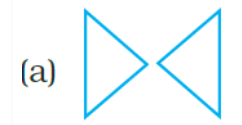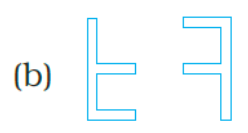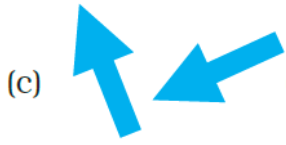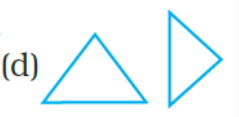Ans: (a)
Explanation: Figure (a) Mirror reflection leads to symmetry, under which the left-right orientation have to be taken care of.

Q.24. Which of these nets is a net of a cube?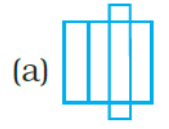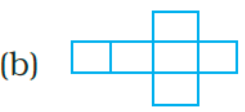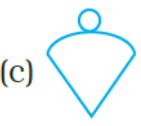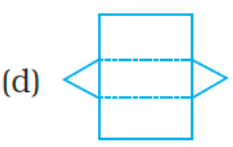Ans: (b)
Explanation: Figure (b) is a net of a cube.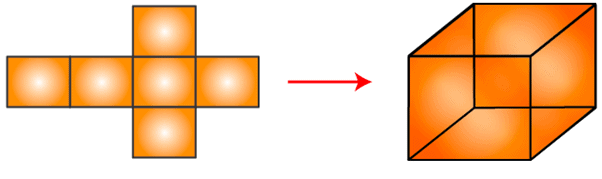Q.25. Which of the following nets is a net of a cylinder?
(a)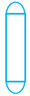(b)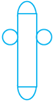(c)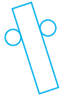(d)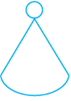Ans: (c)
Explanation: Figure (c) is a net of a cylinder.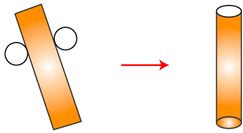Q.26. Which of the following letters of English alphabets have more than 2 lines of symmetry?
(a)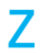(b)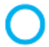(c)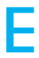(d)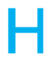Ans: (b)
Explanation: Figure (b) ‘O’ has more than 2 lines of symmetry.

Q.27. Take a square piece of paper as shown in figure (1). Fold it along its diagonals as shown in figure (2). Again fold it as shown in figure (3). Imagine that you have cut off 3 pieces of the form of congruent isosceles right-angled triangles out of it as shown in figure 4.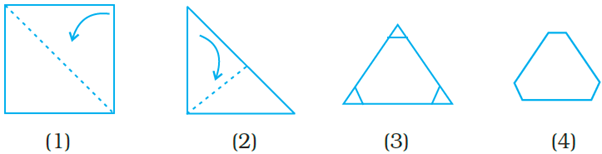On opening the piece of paper which of the following shapes will you get?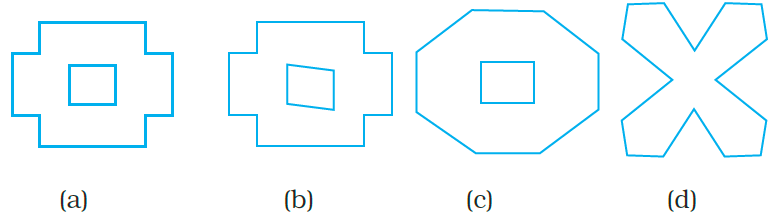Ans: (a)

Q.28. Which of the following 3-dimensional figures has the top, side and front as triangles?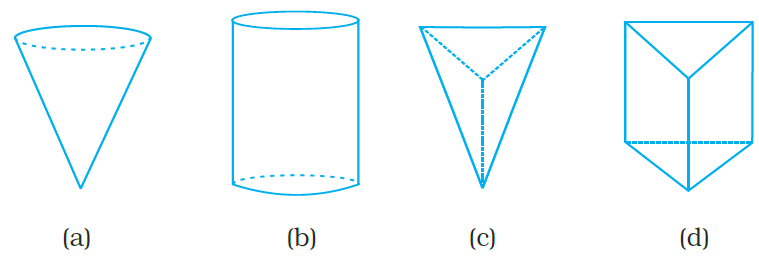Ans: (c)
Explanation: Figure (c) has the top, side and front as triangles.

In Questions 29 to 58, fill in the blanks to make the statements true.
Q.29. In an isosceles right triangle, the number of lines of symmetry is ________.
Solution: In an isosceles right triangle, the number of lines of symmetry is one.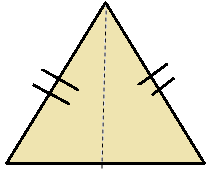Q.30. Rhombus is a figure that has ______lines of symmetry and has a rotational symmetry of order _______.
Solution: Rhombus is a figure that has 2 lines of symmetry and has a rotational symmetry of order 2.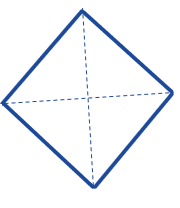Q.31. __________ triangle is a figure that has a line of symmetry but lacks rotational symmetry.
Solution: Isosceles triangle is a figure that has a line of symmetry but lacks rotational symmetry.

Q.32. __________ is a figure that has neither a line of symmetry nor a rotational symmetry.
Solution: Scalene triangle is a figure that has neither a line of symmetry nor a rotational symmetry.

Q.33. __________ and __________ are the capital letters of English alphabets that have one line of symmetry but they interchange to each other when rotated through 180°.
Solution: M and W are the capital letters of English alphabets that have one line of symmetry but they interchange to each other when rotated through 180°.

Q.34. The common portion of two adjacent faces of a cuboid is called __________.
Solution: The common portion of two adjacent faces of a cuboid is called edge.

Q.35. A plane surface of a solid enclosed by edges is called __________ .
Solution: A plane surface of a solid enclosed by edges is called face.

Q.36. The corners of solid shapes are called its __________.
Solution: The corners of solid shapes are called its vertices.

Q.37. A solid with no vertex is __________.
Solution: A solid with no vertex is sphere.

Q.38. A triangular prism has __________ faces, __________ edges and __________ vertices.
Solution:
A triangular prism has 5 faces, 9 edges and 6 vertices.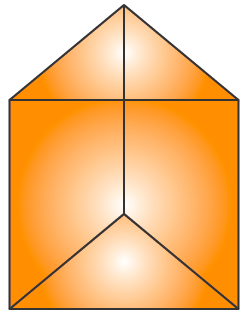Q.39. A triangular pyramid has __________ faces, __________ edges and __________vertices.
Solution:
A triangular pyramid has 4 faces, 6 edges and 4 vertices.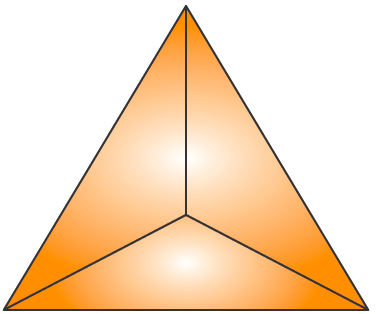Q.40. A square pyramid has __________ faces, __________ edges and __________ vertices.
Solution:
A square pyramid has 5 faces, 8 edges and 5 vertices.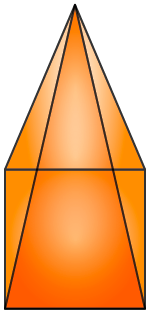Q.41. Out of __________ faces of a triangular prism, __________are rectangles and __________ are triangles.
Solution:
Out of 5 faces of a triangular prism, 3 are rectangles and 2 are triangles.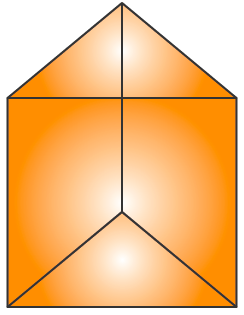Q.42. The base of a triangular pyramid is a __________.
Solution:
The base of a triangular pyramid is a triangle.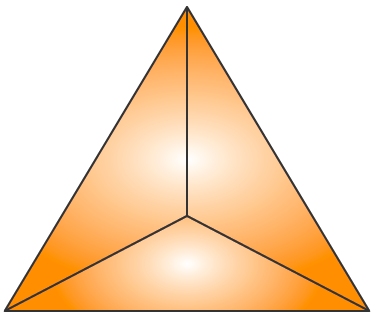Q.43. Out of __________ faces of a square pyramid, __________ are triangles and __________ is/are squares.
Solution:
Out of 5 faces of a square pyramid, 4 are triangles and 1 is/are squares.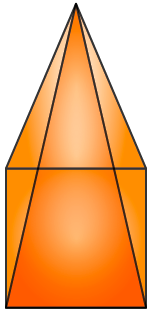Q.44. Out of __________ faces of a rectangular pyramid __________ are triangles and base is __________.
Solution:
Out of 5 faces of a rectangular pyramid 4 are triangles and base is rectangle.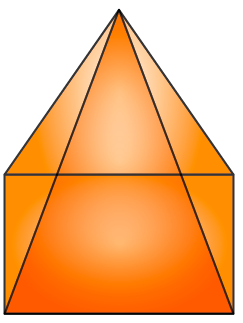Q.45. Each of the letters H, N, S and Z has a rotational symmetry of order __________.
Solution:
Each of the letters H, N, S and Z has a rotational symmetry of order 2.

Q.46. Order of rotational symmetry of a rectangle is __________.
Solution:
Order of rotational symmetry of a rectangle is 2. In a complete turn (of 360°), the number of times the figure coincides with its original position is called its order of rotational symmetry.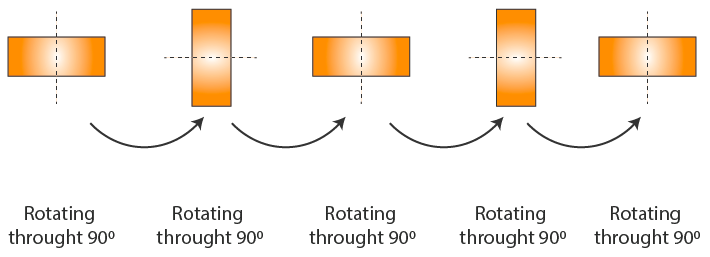Q.47. Order of rotational symmetry of a circle is __________.
Solution:
Order of rotational symmetry of a circle is 2. In a complete turn (of 360°), the number of times the figure coincides with its original position is called its order of rotational symmetry.

Q.48. Each face of a cuboid is a __________.
Solution:
Each face of a cuboid is a rectangle.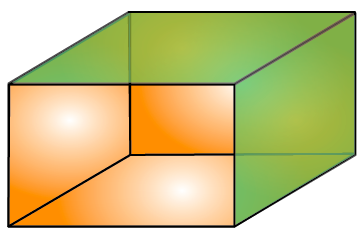Q.49. Line of symmetry for an angle is its __________.
Solution:
Line of symmetry for an angle is its bisector.

Q.50. A parallelogram has __________ line of symmetry.
Solution:
A parallelogram has no line of symmetry.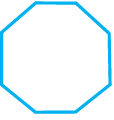Q.51. Order of rotational symmetry of is.
Solution: Order of rotational symmetry of given figure is 8.
We know that, in a complete turn (of 360°), the number of times the figure coincides with its original position is called its order of rotational symmetry.

Q.52. A __________ triangle has no lines of symmetry.
Solution: A scalene triangle has no lines of symmetry.
We know that, in scalene triangle all angles and sides are unequal.

Q.53. Cuboid is a rectangular_________.
Solution: Cuboid is a rectangular prism.

Q.54. A sphere has __________vertex, __________edge and __________curved surface.
Solution: A sphere has 0 vertex, 0 edge and 1 curved surface.

Q.55.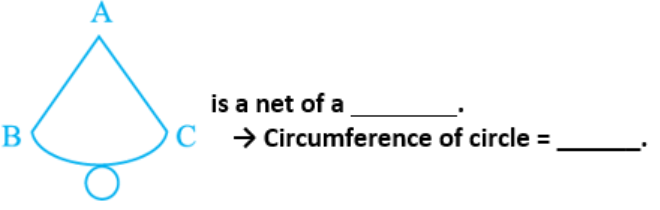Solution: The given figure is net of cone.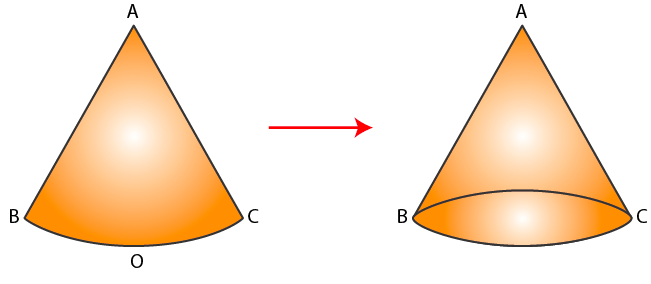Circumference of circle 2πr.

Q.56.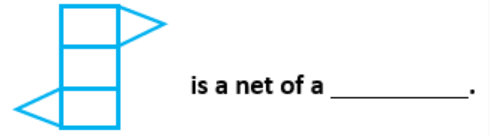Solution: The given figure is a net of triangular prism.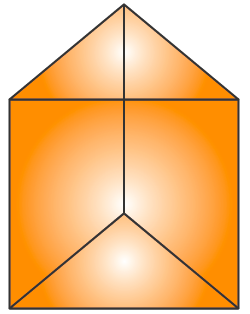Q.57.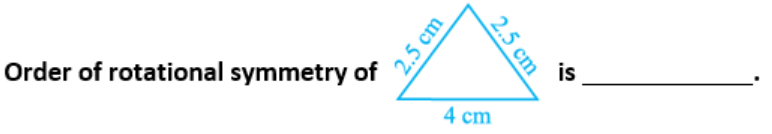Solution: Order of rotational symmetry of given figure is 1.

Q.58. Identical cubes are stacked in the corner of a room as shown below. The number of cubes that are not visible are _________.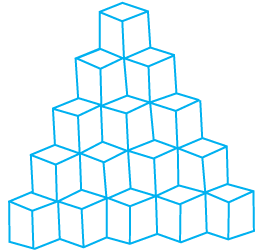Solution: The number of cubes that are not visible are 20.

In Questions from 59 to 62, state whether the statements are True or False.
Q.59. We can draw exactly one triangle whose angles are 70°, 30° and 80°.
Solution: False.
By using the given angles we can able to draw more number of triangles.

Q.60. The distance between the two parallel lines is the same everywhere.
Solution: True.

Q.61. A circle has two lines of symmetry.
Solution: False.
Circle has infinite lines of symmetry.

Q.62. An angle has two lines of symmetry
Solution: False.
An angle has one line of symmetry.

The document NCERT Exemplar Solutions: Practical Geometry Symmetry & Visualising Solid Shapes Notes | Study Mathematics (Maths) Class 7 - Class 7 is a part of the Class 7 Course Mathematics (Maths) Class 7.
All you need of Class 7 at this link: Class 7Use Code STAYHOME200 and get INR 200 additional OFF Use Coupon Code

Top Courses for Class 7Mathematics (Maths) Class 7

161 videos|237 docs|45 tests

Top Courses for Class 7Track your progress, build streaks, highlight & save important lessons and more!

,

,

,

,

,

,

,

,

,

,

,

,

,

,

,

,

,

,

,

,

,

;